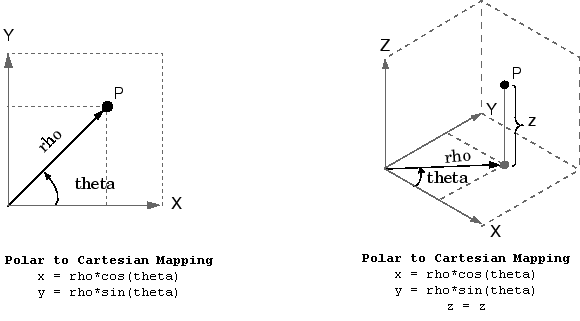# pol2cart

## 语法

``[x,y] = pol2cart(theta,rho)``
``[x,y,z] = pol2cart(theta,rho,z)``

## 说明

``[x,y] = pol2cart(theta,rho)` 将极坐标数组 `theta` 和 `rho` 的对应元素变换为二维笛卡尔坐标或 xy 坐标。`

``[x,y,z] = pol2cart(theta,rho,z)` 将柱坐标数组 `theta`、`rho` 和 `z` 的对应元素变换为三维笛卡尔坐标或 xyz 坐标。`

## 示例

`theta = [0 pi/4 pi/2 pi]`
```theta = 1×4 0 0.7854 1.5708 3.1416 ```
`rho = [5 5 10 10]`
```rho = 1×4 5 5 10 10 ```
`[x,y] = pol2cart(theta,rho)`
```x = 1×4 5.0000 3.5355 0.0000 -10.0000 ```
```y = 1×4 0 3.5355 10.0000 0.0000 ```

`theta = [0 pi/4 pi/2 pi]'`
```theta = 4×1 0 0.7854 1.5708 3.1416 ```
`rho = [1 3 4 5]'`
```rho = 4×1 1 3 4 5 ```
`z = [7 8 9 10]'`
```z = 4×1 7 8 9 10 ```
`[x,y,z] = pol2cart(theta,rho,z)`
```x = 4×1 1.0000 2.1213 0.0000 -5.0000 ```
```y = 4×1 0 2.1213 4.0000 0.0000 ```
```z = 4×1 7 8 9 10 ```

## 输入参数

`theta` 是在 x-y 平面上从 x 轴的正向测量的逆时针角度（以弧度为单位）。

`rho` 是从原点到 x-y 平面上某点的距离。

`z` 是高出 x-y 平面的高度。

## 算法